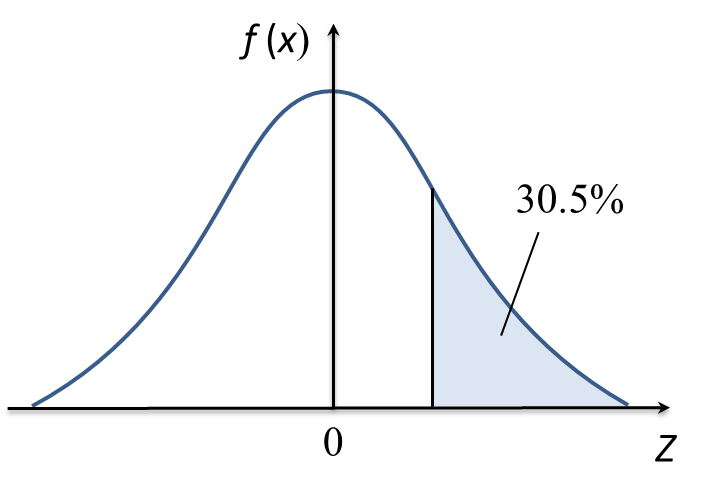# 5.4.3 Probability Distributions, Long Questions

Question 8:
(a) A survey is carried out about red crescents in a school.
It is found that the mean of the number of red crescents is 315, the variance is 126 and the probability that a student participate in red crescents is p.
(i) Find the value of p.
(ii) If 8 students from the school are chosen at random, find the probability that more than 5 students participate in red crescents.

(b) The mass of fertiliser used in an orchard farm is normally distributed with mean 5 kg and variance 0.8 kg. Find the probability that on a particular day, more than 6 kg of fertiliser is used.

Solution:

Question 9:
(a) 30% of the pens in a box are blue. Charlie picks 4 pens at random. Find the probability that at least one pen picked is not blue.

(b) The mass of papayas harvested from an orchard farm follows a normal distribution with a mean of 2 kg and a standard deviation of h kg. It is given that 15.87% of the papayas have a mass more than 2.5 kg.
(i) Calculate the value of h.
(ii) Given the number of papayas harvested from the orchard farm is 1320, find the number of papayas that have the mass between 1.0 kg and 2.5 kg.

Solution:Question 10:
(a) It is found that 60% of the students from a certain class obtained grade A in English in O level trial examination.
If 10 students from the class are selected at random, find the probability that
(i) exactly 7 students obtained grade A.
(ii) not more than 7 students obtained grade A.

(b) Diagram below shows a standard normal distribution graph representing the volume of soy sauce in bottles produced by a factory.It is given the mean is 950 cm3 and the variance is 256 cm6. If the percentage of the volume more than V is 30.5%, find
(i) the value of V,
(ii) the probability that the volume between 930 cm3 and 960 cm3.

Solution:

$\begin{array}{l}\left(ii\right)\\ \text{Probability}\\ =P\left(9301.25\right)-P\left(Z>0.625\right)\\ =1-0.1056-0.2660\\ =0.6284\end{array}$